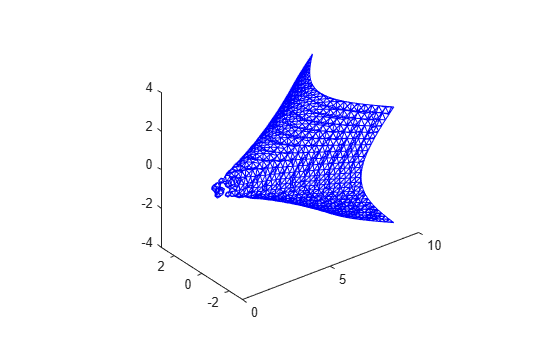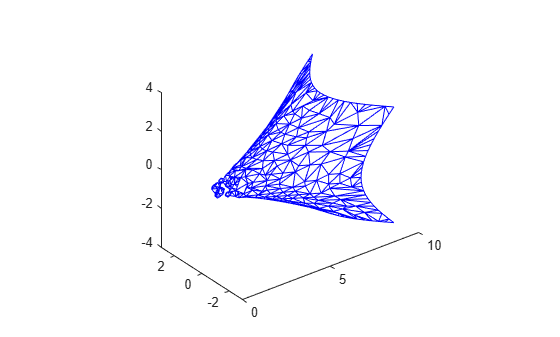# reducepatch

Reduce number of patch faces

## Syntax

```reducepatch(p,r) nfv = reducepatch(p,r) nfv = reducepatch(fv,r) nfv = reducepatch(p) nfv = reducepatch(fv) reducepatch(...,'fast') reducepatch(...,'verbose') nfv = reducepatch(f,v,r) [nf,nv] = reducepatch(...) ```

## Description

`reducepatch(p,r)` reduces the number of faces of the patch identified by handle `p`, while attempting to preserve the overall shape of the original object. The MATLAB® software interprets the reduction factor `r` in one of two ways depending on its value:

• If `r` is less than 1, `r` is interpreted as a fraction of the original number of faces. For example, if you specify `r` as 0.2, then the number of faces is reduced to 20% of the number in the original patch.

• If `r` is greater than or equal to 1, then `r` is the target number of faces. For example, if you specify `r` as 400, then the number of faces is reduced until there are 400 faces remaining.

`nfv = reducepatch(p,r)` returns the reduced set of faces and vertices but does not set the `Faces` and `Vertices` properties of patch `p`. The struct `nfv` contains the faces and vertices after reduction.

`nfv = reducepatch(fv,r)` performs the reduction on the faces and vertices in the struct `fv`.

`nfv = reducepatch(p)` and ```nfv = reducepatch(fv)```uses a reduction value of `0.5`.

`reducepatch(...,'fast')` assumes the vertices are unique and does not compute shared vertices.

`reducepatch(...,'verbose')` prints progress messages to the command window as the computation progresses.

`nfv = reducepatch(f,v,r)`performs the reduction on the faces in `f` and the vertices in `v`.

`[nf,nv] = reducepatch(...)` returns the faces and vertices in the arrays `nf` and `nv`.

## Examples

collapse all

This example illustrates the effect of reducing the number of faces to only 15% of the original value.

```figure [x,y,z,v] = flow; p = patch(isosurface(x,y,z,v,-3)); p.FaceColor = 'w'; p.EdgeColor = 'b'; daspect([1,1,1]) view(3)```Reduce the number of faces.

`reducepatch(p,0.15)`## Tips

If the patch contains nonshared vertices, MATLAB computes shared vertices before reducing the number of faces. If the faces of the patch are not triangles, MATLAB triangulates the faces before reduction. The faces returned are always defined as triangles.

The number of output triangles may not be exactly the number specified with the reduction factor argument (`r`), particularly if the faces of the original patch are not triangles.